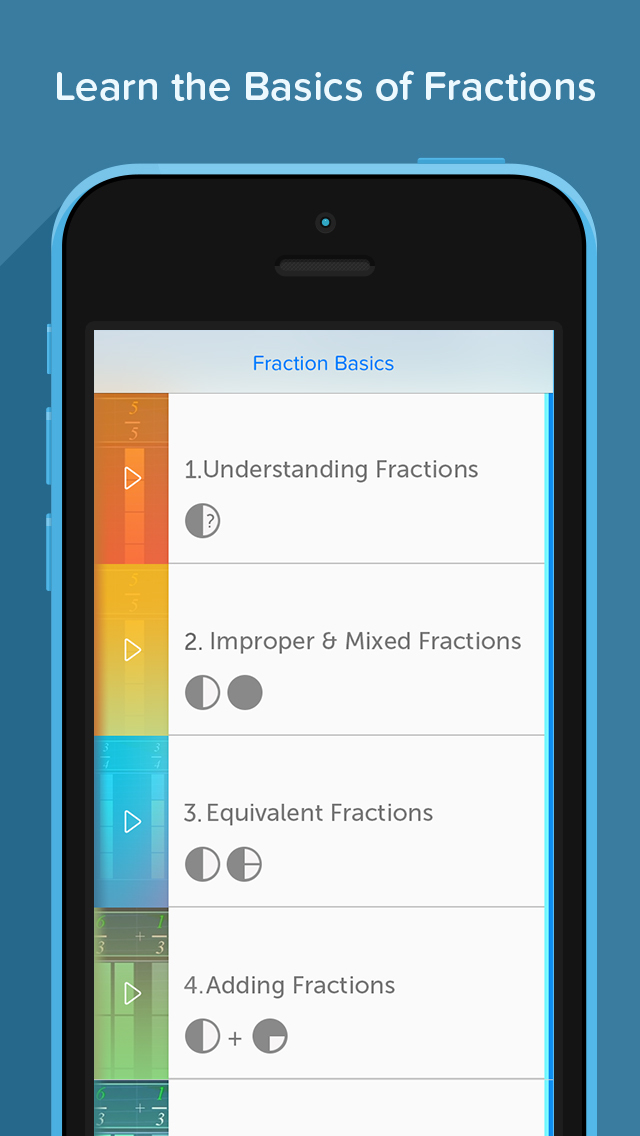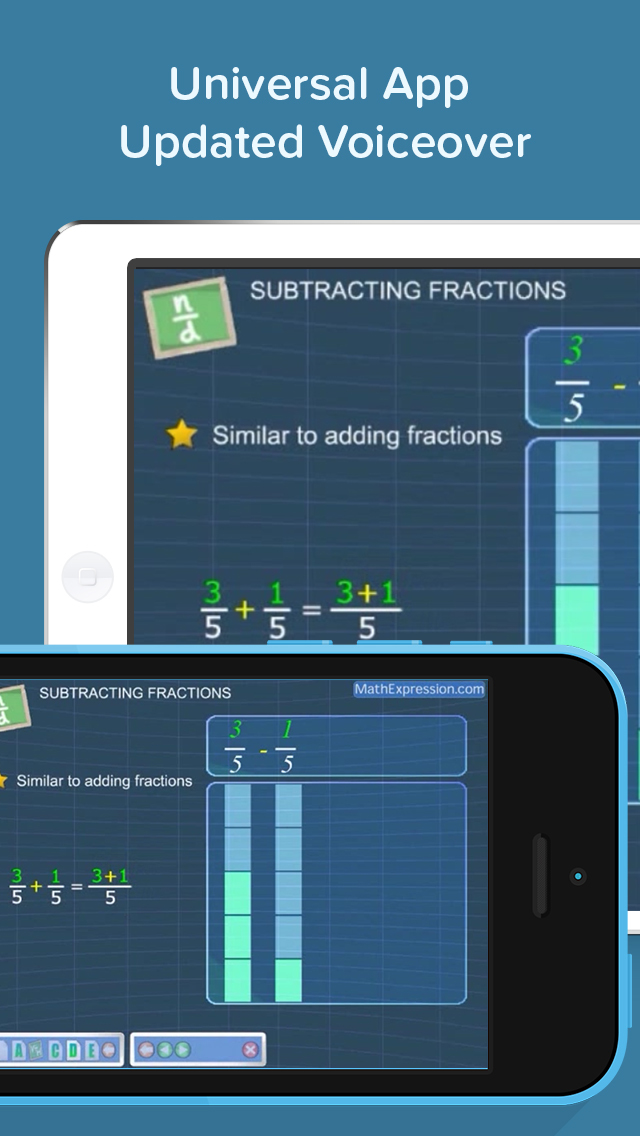# Fraction Basics - Easy & Effective Fractions Tutor - Lumos Educational App Store41
Price - Free
\$0

#### DESCRIPTION:

Hurray!! Thanks to our awesome engineers for swift action. If youÃÂ¢Ã¢âÂ¬Ã¢âÂ¢re struggling with fraction problems or looking for a quick, smart and effective way to master the basics, Fraction Basics is your answer. This free video app teaches you the core concepts of fractions in 12 computer-animated, visually interactive videos. Fraction Basics is designed to help you easily navigate the videos to access just the lesson you need to tackle, and you can easily skip to your problem areas or targeted sections. Our video app is divided into the following parts liste

#### OVERVIEW:

Fraction Basics - Easy & Effective Fractions Tutor is a free educational mobile app By Visual Math Interactive Sdn. Bhd..It helps students in grades 3,4,5 practice the following standards 3.NF.1,4.NF.1,5.NF.1.

This page not only allows students and teachers download Fraction Basics - Easy & Effective Fractions Tutor but also find engaging Sample Questions, Videos, Pins, Worksheets, Books related to the following topics.

1. 3.NF.1 : Understand a fraction 1/b as the quantity formed by 1 part when a whole is partitioned into b equal parts; understand a fraction a/b as the quantity formed by a parts of size 1/b. (Grade 3 expectations in this domain are limited to fractions with denominators 2, 3, 4, 6, and 8.).

2. 4.NF.1 : Explain why a fraction a/b is equivalent to a fraction (n .

3. 5.NF.1 : Add and subtract fractions with unlike denominators (including mixed numbers) by replacing given fractions with equivalent fractions in such a way as to produce an equivalent sum or difference of fractions with like denominators. For example, 2/3 + 5/4 = 8/12 + 15/12 = 23/12. (In general, a/b + c/d = (ad + bc)/bd.) .

3
4
5

#### STANDARDS:

3.NF.1
4.NF.1
5.NF.1

Developer: Visual Math Interactive Sdn. Bhd.

Software Version: 2.8

Category: Education

Release Date: 2010-11-24T21:19:43Z### RELATED APPSEdSearch WebSearch